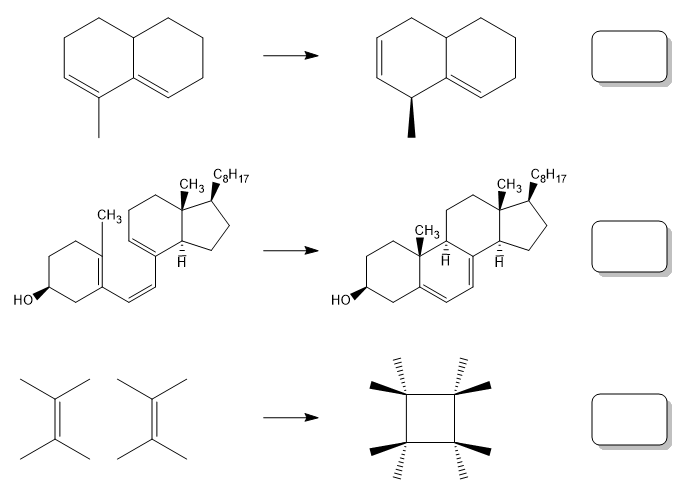Problem: Identify the following pericyclic reactions. Use the following letters to indicate if the reaction is a cycloaddition (C), electrocyclic reaction (E) or sigmatropic shift (S).

FREE Expert Solution
88% (246 ratings)
Problem Details

Identify the following pericyclic reactions. Use the following letters to indicate if the reaction is a cycloaddition (C), electrocyclic reaction (E) or sigmatropic shift (S).What scientific concept do you need to know in order to solve this problem?

Our tutors have indicated that to solve this problem you will need to apply the Pericyclic Reaction concept. You can view video lessons to learn Pericyclic Reaction Or if you need more Pericyclic Reaction practice, you can also practice Pericyclic Reaction practice problems .

What is the difficulty of this problem?

Our tutors rated the difficulty of Identify the following pericyclic reactions. Use the followi... as low difficulty.

How long does this problem take to solve?

Our expert Organic tutor, Johnny took 3 minutes to solve this problem. You can follow their steps in the video explanation above.

What professor is this problem relevant for?

Based on our data, we think this problem is relevant for Professor Cruz's class at USF.